# http www comp nus edu sgcs 1010 UNIT

• Slides: 15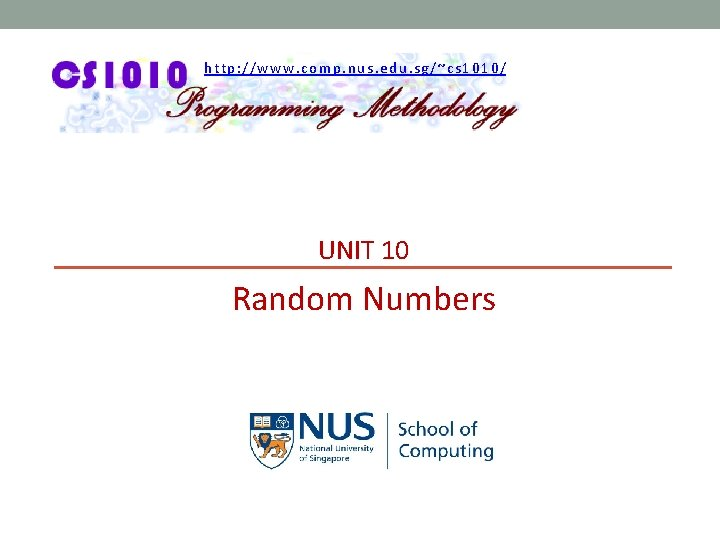http: //www. comp. nus. edu. sg/~cs 1010/ UNIT 10 Random NumbersAaron Tan, NUS Random Numbers Unit 10: Random Numbers Objective: § Learn the use of random numbers Unit 10 - 2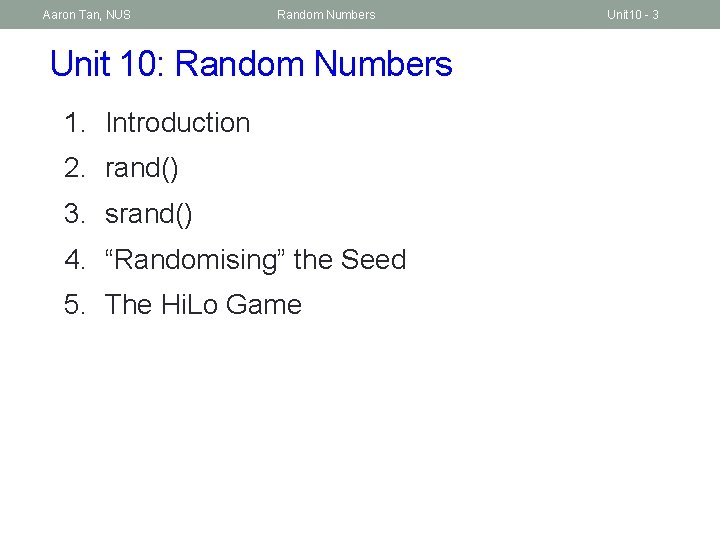Aaron Tan, NUS Random Numbers Unit 10: Random Numbers 1. Introduction 2. rand() 3. srand() 4. “Randomising” the Seed 5. The Hi. Lo Game Unit 10 - 3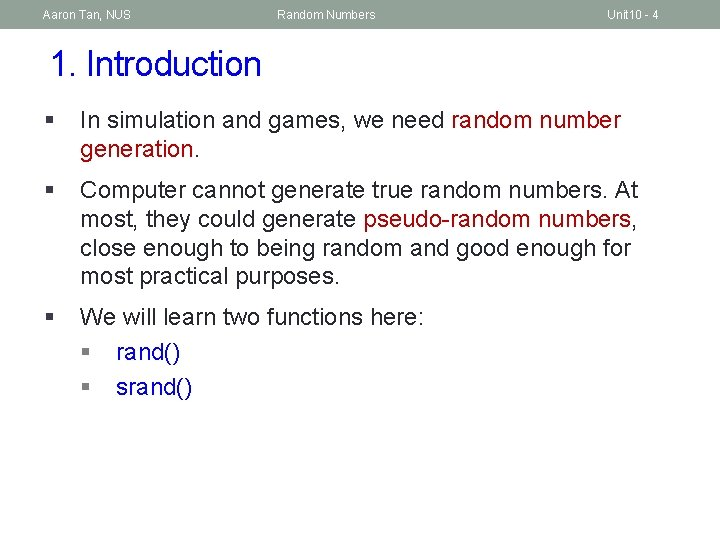Aaron Tan, NUS Random Numbers Unit 10 - 4 1. Introduction § In simulation and games, we need random number generation. § Computer cannot generate true random numbers. At most, they could generate pseudo-random numbers, close enough to being random and good enough for most practical purposes. § We will learn two functions here: § rand() § srand()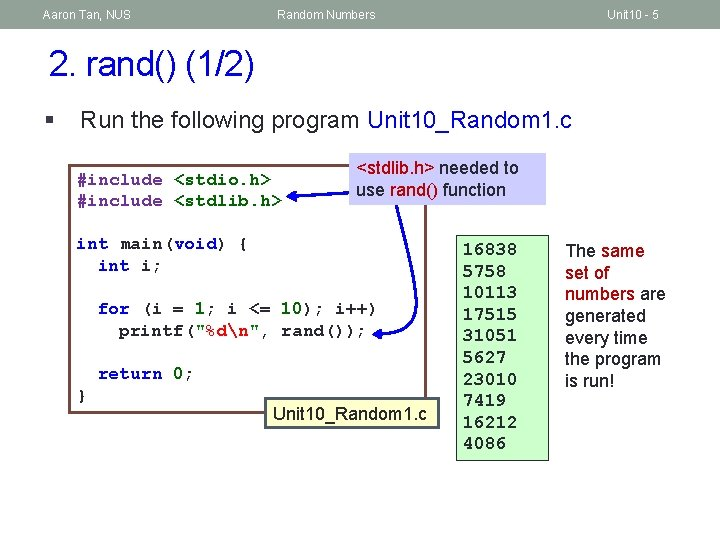Aaron Tan, NUS Random Numbers Unit 10 - 5 2. rand() (1/2) § Run the following program Unit 10_Random 1. c #include <stdio. h> #include <stdlib. h> needed to use rand() function int main(void) { int i; for (i = 1; i <= 10); i++) printf("%dn", rand()); return 0; } Unit 10_Random 1. c 16838 5758 10113 17515 31051 5627 23010 7419 16212 4086 The same set of numbers are generated every time the program is run!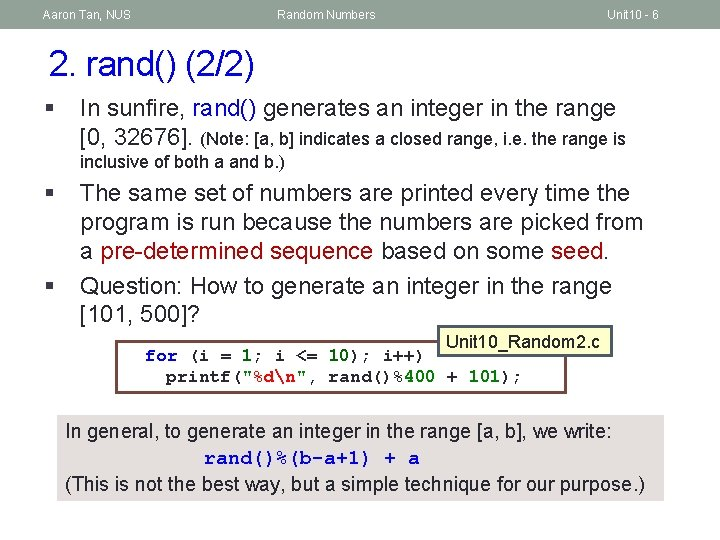Aaron Tan, NUS Random Numbers Unit 10 - 6 2. rand() (2/2) § In sunfire, rand() generates an integer in the range [0, 32676]. (Note: [a, b] indicates a closed range, i. e. the range is inclusive of both a and b. ) § § The same set of numbers are printed every time the program is run because the numbers are picked from a pre-determined sequence based on some seed. Question: How to generate an integer in the range [101, 500]? Unit 10_Random 2. c for (i = 1; i <= 10); i++) printf("%dn", rand()%400 + 101); In general, to generate an integer in the range [a, b], we write: rand()%(b-a+1) + a (This is not the best way, but a simple technique for our purpose. )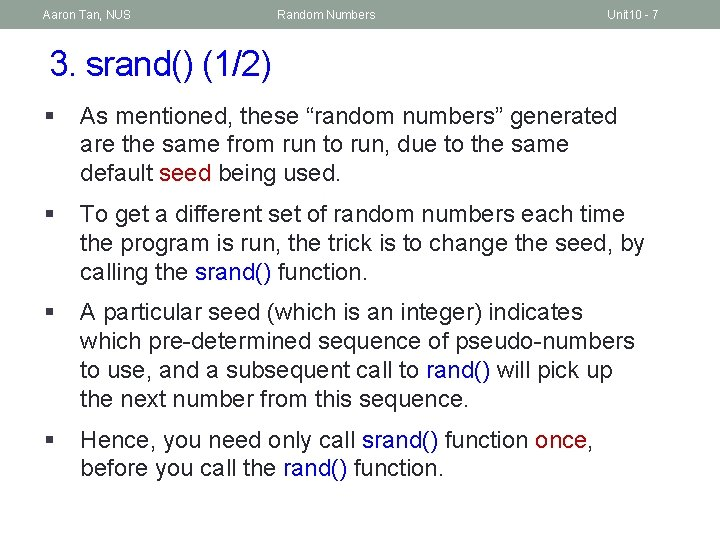Aaron Tan, NUS Random Numbers Unit 10 - 7 3. srand() (1/2) § As mentioned, these “random numbers” generated are the same from run to run, due to the same default seed being used. § To get a different set of random numbers each time the program is run, the trick is to change the seed, by calling the srand() function. § A particular seed (which is an integer) indicates which pre-determined sequence of pseudo-numbers to use, and a subsequent call to rand() will pick up the next number from this sequence. § Hence, you need only call srand() function once, before you call the rand() function.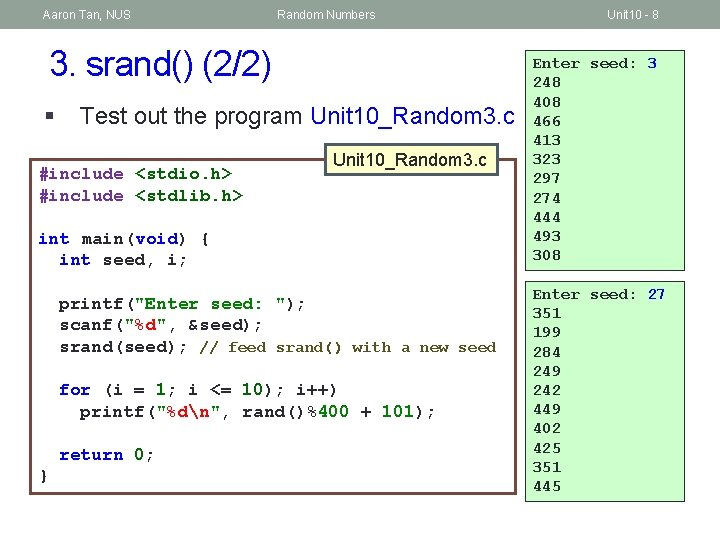Aaron Tan, NUS Random Numbers 3. srand() (2/2) § Test out the program Unit 10_Random 3. c #include <stdio. h> #include <stdlib. h> Unit 10_Random 3. c int main(void) { int seed, i; printf("Enter seed: "); scanf("%d", &seed); srand(seed); // feed srand() with a new seed for (i = 1; i <= 10); i++) printf("%dn", rand()%400 + 101); return 0; } Unit 10 - 8 Enter seed: 3 248 408 466 413 323 297 274 444 493 308 Enter seed: 27 351 199 284 249 242 449 402 425 351 445Aaron Tan, NUS Random Numbers Unit 10 - 9 4. “Randomising” the Seed (1/2) § § In the preceding example, the user is asked to enter a value for the seed. However, in many applications such as games or simulations, we want to “automate” this step since we do not want user’s invention. How do we ensure that every time the program is run, a different seed is used? One simple solution is to use the time(NULL) function, which returns an integer that is the number of seconds since 1 st of January 1970. This value can then be used as the seed for the srand() function.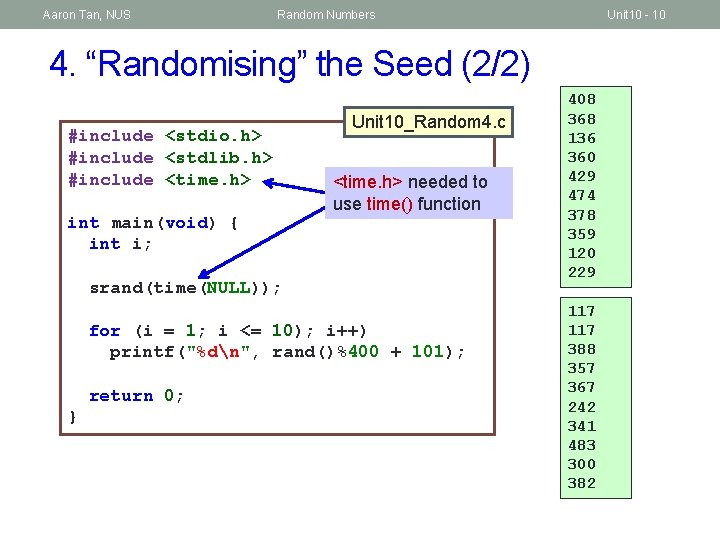Aaron Tan, NUS Random Numbers Unit 10 - 10 4. “Randomising” the Seed (2/2) #include <stdio. h> #include <stdlib. h> #include <time. h> int main(void) { int i; Unit 10_Random 4. c <time. h> needed to use time() function srand(time(NULL)); for (i = 1; i <= 10); i++) printf("%dn", rand()%400 + 101); return 0; } 408 368 136 360 429 474 378 359 120 229 117 388 357 367 242 341 483 300 382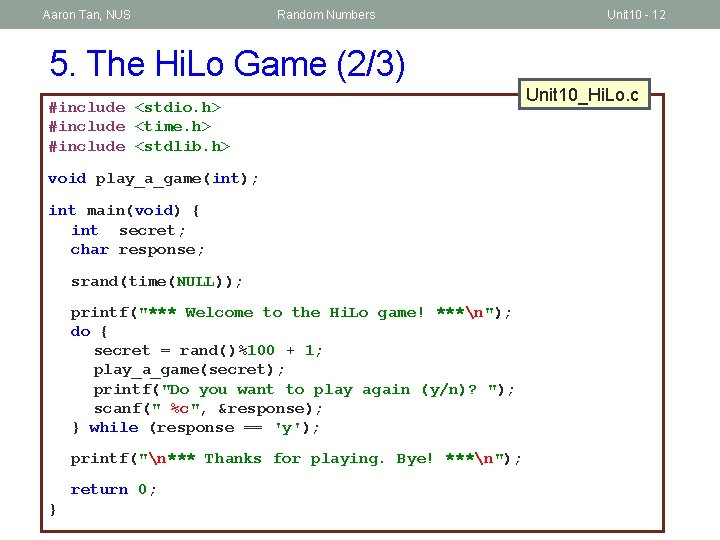Aaron Tan, NUS Random Numbers Unit 10 - 12 5. The Hi. Lo Game (2/3) #include <stdio. h> #include <time. h> #include <stdlib. h> void play_a_game(int); int main(void) { int secret; char response; srand(time(NULL)); printf("*** Welcome to the Hi. Lo game! ***n"); do { secret = rand()%100 + 1; play_a_game(secret); printf("Do you want to play again (y/n)? "); scanf(" %c", &response); } while (response == 'y'); printf("n*** Thanks for playing. Bye! ***n"); return 0; } Unit 10_Hi. Lo. c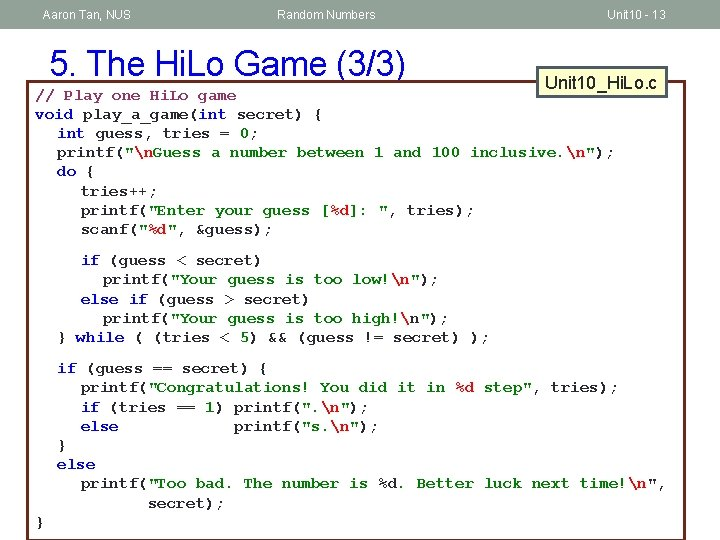Aaron Tan, NUS Random Numbers 5. The Hi. Lo Game (3/3) Unit 10 - 13 Unit 10_Hi. Lo. c // Play one Hi. Lo game void play_a_game(int secret) { int guess, tries = 0; printf("n. Guess a number between 1 and 100 inclusive. n"); do { tries++; printf("Enter your guess [%d]: ", tries); scanf("%d", &guess); if (guess < secret) printf("Your guess is too low!n"); else if (guess > secret) printf("Your guess is too high!n"); } while ( (tries < 5) && (guess != secret) ); if (guess == secret) { printf("Congratulations! You did it in %d step", tries); if (tries == 1) printf(". n"); else printf("s. n"); } else printf("Too bad. The number is %d. Better luck next time!n", secret); }Aaron Tan, NUS Random Numbers Unit 10 - 14 Summary n In this unit, you have learned about n Generating pseudo-random numbers using rand() n Seeding a pseudo-random sequence using srand() n Providing a “random” seed by using time(NULL) in the srand() function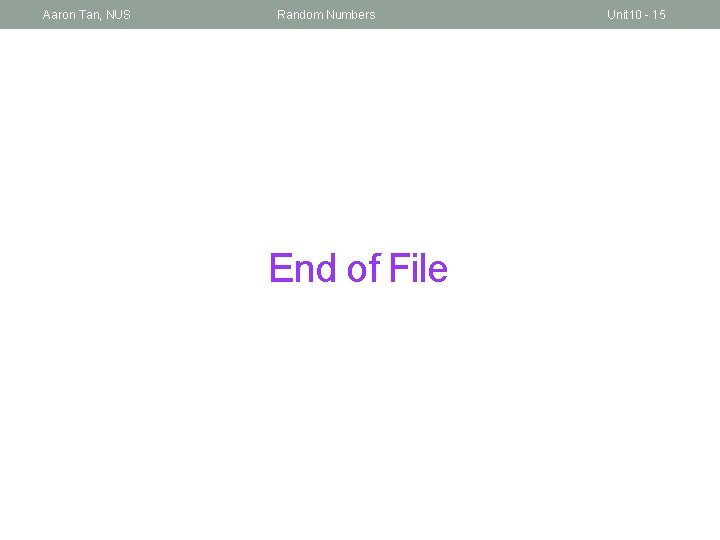Aaron Tan, NUS Random Numbers End of File Unit 10 - 15